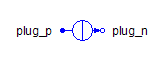Shuttle Valve - MapleSim Help

Shuttle Valve

Shuttle valve with pressure biasDescription

The Shuttle Valve component allows flow out of port C from the either port A or port B, depending which has the higher pressure.

Based on the orifice area, the pressure vs. flow rate relationship is calculated by the formulation used in the Orifice component.Formulation Approaches One of two approaches can be selected for modeling the flow in the device. When the boolean parameter $\mathrm{Use constant Cd}$ is true, a constant coefficient of discharge (${C}_{d}$) is used, otherwise a variable coefficient of discharge with maximum value (${C}_{d\left(\mathrm{max}\right)}$) and a critical flow number (${\mathrm{Crit}}_{\mathrm{no}}$) are used.Optional Volumes The boolean parameters $\mathrm{Use volume A}$, $\mathrm{Use volume B}$, and $\mathrm{Use volume C}$ when true, add optional volumes ${V}_{A}$, ${V}_{B}$, and ${V}_{C}$ to ports A, B, and C, respectively. See Port Volumes for details. If two orifices or valves are connected, enabling a volume at the common port reduces the stiffness of the system and improves the solvability.Equations ${p}_{\mathrm{AB}}={p}_{A}-{p}_{B}\phantom{\rule[-0.0ex]{4.5ex}{0.0ex}}{P}_{\mathrm{BC}}={p}_{B}-{p}_{C}$ ${q}_{\mathrm{AA}}={q}_{A}-{q}_{{V}_{A}}\phantom{\rule[-0.0ex]{4.5ex}{0.0ex}}{q}_{\mathrm{BB}}={q}_{B}-{q}_{{V}_{B}}\phantom{\rule[-0.0ex]{4.5ex}{0.0ex}}{q}_{\mathrm{CC}}={q}_{C}-{q}_{{V}_{C}}$ ${q}_{\mathrm{AA}}+{q}_{\mathrm{BB}}+\mathrm{qCC}=0$ $\mathbf{Orifice Fluid Equations}$ $\left\{\begin{array}{cc}{p}_{\mathrm{AC}}=\frac{\mathrm{\pi }}{4}\frac{\mathrm{\rho }\mathrm{\nu }{q}_{\mathrm{AA}}}{{C}_{d}^{2}{A}_{{\mathrm{cs}}_{1}}\sqrt{\mathrm{\pi }{A}_{{\mathrm{cs}}_{1}}}}{\left(\frac{16{q}_{\mathrm{AA}}^{4}}{{\mathrm{\pi }}^{2}{A}_{{\mathrm{cs}}_{1}}^{2}{\mathrm{\nu }}^{4}}+{\mathrm{Re}}_{\mathrm{Cr}}^{4}\right)}^{\frac{1}{4}}& \mathrm{Use constant Cd}=\mathrm{true}\\ {q}_{\mathrm{AA}}={C}_{d\left(\mathrm{max}\right)}\mathrm{tanh}\left(4\frac{\sqrt{\frac{{A}_{{\mathrm{cs}}_{1}}}{\mathrm{\pi }}\frac{2\left|{p}_{\mathrm{AC}}\right|}{\mathrm{\rho }}}}{\mathrm{\nu }{\mathrm{Crit}}_{\mathrm{no}}}\right){A}_{{\mathrm{cs}}_{1}}\sqrt{\frac{2\left|{p}_{\mathrm{AC}}\right|}{\mathrm{\rho }}}\mathrm{sign}\left({p}_{\mathrm{AC}}\right)& \mathrm{otherwise}\end{array}$ $\left\{\begin{array}{cc}{p}_{\mathrm{BC}}=\frac{\mathrm{\pi }}{4}\frac{\mathrm{\rho }\mathrm{\nu }{q}_{\mathrm{BB}}}{{C}_{d}^{2}{A}_{{\mathrm{cs}}_{2}}\sqrt{\mathrm{\pi }{A}_{{\mathrm{cs}}_{2}}}}{\left(\frac{16{q}_{\mathrm{BB}}^{4}}{{\mathrm{\pi }}^{2}{A}_{{\mathrm{cs}}_{2}}^{2}{\mathrm{\nu }}^{4}}+{\mathrm{Re}}_{\mathrm{Cr}}^{4}\right)}^{\frac{1}{4}}& \mathrm{Use constant Cd}=\mathrm{true}\\ {q}_{\mathrm{BB}}={C}_{d\left(\mathrm{max}\right)}\mathrm{tanh}\left(4\frac{\sqrt{\frac{{A}_{{\mathrm{cs}}_{2}}}{\mathrm{\pi }}\frac{2\left|{p}_{\mathrm{BC}}\right|}{\mathrm{\rho }}}}{\mathrm{\nu }{\mathrm{Crit}}_{\mathrm{no}}}\right){A}_{{\mathrm{cs}}_{2}}\sqrt{\frac{2\left|{p}_{\mathrm{BC}}\right|}{\mathrm{\rho }}}\mathrm{sign}\left({p}_{\mathrm{BC}}\right)& \mathrm{otherwise}\end{array}$ $\left\{\begin{array}{cc}\left\{{A}_{{\mathrm{cs}}_{1}}={A}_{i}={A}_{t}\right\}& \mathrm{Exact}\\ \left\{{A}_{{\mathrm{cs}}_{1}}=\mathrm{min}\left({A}_{\mathrm{open}},\mathrm{max}\left({A}_{\mathrm{close}},{A}_{i}\right)\right),{t}_{c}\frac{\mathrm{d}{A}_{i}}{\mathrm{d}t}+{A}_{i}={A}_{t}\right\}& \mathrm{otherwise}\end{array}$ ${A}_{{\mathrm{cs}}_{2}}={A}_{\mathrm{open}}+{A}_{\mathrm{close}}-{A}_{{\mathrm{cs}}_{1}}$ ${A}_{t}=\frac{1}{2}\left({A}_{\mathrm{open}}-{A}_{\mathrm{close}}\right)\left(1+\mathrm{tanh}\left(\mathrm{eps}\cdot \left({p}_{\mathrm{AB}}-{p}_{\mathrm{open}}\right)\right)\right)$ $\mathbf{Optional Volume Equations}$ ${V}_{{f}_{A}}=\left\{\begin{array}{cc}\mathrm{Va}\left(1+\frac{{p}_{A}}{\mathrm{El}}\right)& \mathrm{Use volume A}=\mathrm{true}\\ 0& \mathrm{otherwise}\end{array}\phantom{\rule[-0.0ex]{5.0ex}{0.0ex}}{q}_{{V}_{A}}=\left\{\begin{array}{cc}\frac{\mathrm{d}{V}_{{f}_{A}}}{\mathrm{d}t}& \mathrm{Use volume A}=\mathrm{true}\\ 0& \mathrm{otherwise}\end{array}$ ${V}_{{f}_{B}}=\left\{\begin{array}{cc}\mathrm{Vb}\left(1+\frac{{p}_{B}}{\mathrm{El}}\right)& \mathrm{Use volume B}=\mathrm{true}\\ 0& \mathrm{otherwise}\end{array}\phantom{\rule[-0.0ex]{5.0ex}{0.0ex}}{q}_{{V}_{B}}=\left\{\begin{array}{cc}\frac{\mathrm{d}{V}_{{f}_{B}}}{\mathrm{d}t}& \mathrm{Use volume B}=\mathrm{true}\\ 0& \mathrm{otherwise}\end{array}$ ${V}_{{f}_{C}}=\left\{\begin{array}{cc}\mathrm{Vb}\left(1+\frac{{p}_{C}}{\mathrm{El}}\right)& \mathrm{Use volume C}=\mathrm{true}\\ 0& \mathrm{otherwise}\end{array}\phantom{\rule[-0.0ex]{5.0ex}{0.0ex}}{q}_{{V}_{C}}=\left\{\begin{array}{cc}\frac{\mathrm{d}{V}_{{f}_{C}}}{\mathrm{d}t}& \mathrm{Use volume C}=\mathrm{true}\\ 0& \mathrm{otherwise}\end{array}$Variables

 Name Units Description Modelica ID ${p}_{X}$ $\mathrm{Pa}$ Pressure at port X pX ${p}_{\mathrm{XY}}$ $\mathrm{Pa}$ Pressure drop from X to Y pXY ${q}_{\mathrm{XX}}$ $\frac{{m}^{3}}{s}$ Flow rate into port X qXX ${q}_{{V}_{X}}$ $\frac{{m}^{3}}{s}$ Flow rate into port X's optional volume qVX ${V}_{{f}_{X}}$ ${m}^{3}$ Effective volume at port X VfX ${A}_{{\mathrm{cs}}_{1}}$ ${m}^{2}$ Cross-sectional area from A to B Acs ${A}_{{\mathrm{cs}}_{2}}$ ${m}^{2}$ Cross-sectional area from B to C Acs ${A}_{i}$ ${m}^{2}$ Filtered interpolated area Ai ${A}_{t}$ ${m}^{2}$ Interpolated area At ${u}_{1}$ $\frac{m}{s}$ Fluid velocity from A to B u ${u}_{2}$ $\frac{m}{s}$ Fluid velocity from B to C u

$X,Y\in \left\{A,B,C\right\}$Connections

 Name Description Modelica ID $\mathrm{portA}$ Hydraulic port of left inlet portA $\mathrm{portB}$ Hydraulic port of right inlet portB $\mathrm{portC}$ Hydraulic port of bottom outlet portCParametersGeneral

 Name Default Units Description Modelica ID ${p}_{\mathrm{open}}$ $1·{10}^{4}$ $\mathrm{Pa}$ Pressure difference to make one side fully open popen ${A}_{\mathrm{close}}$ $1·{10}^{-12}$ ${m}^{2}$ Orifice area when closed (leakage) Aclose ${A}_{\mathrm{open}}$ $1·{10}^{-5}$ ${m}^{2}$ Orifice area when fully open Aopen $\mathrm{eps}$ $0.2$ $\frac{1}{\mathrm{Pa}}$ Positive factor that effects transition between ports A and B eps $\mathrm{Exact}$ $\mathrm{false}$ True (checked) means first-order dynamics are used for the valve area Exact ${t}_{c}$ $0.1$ $s$ Time constant tcOrifice

 Name Default Units Description Modelica ID $\mathrm{Use constant Cd}$ $\mathrm{true}$ True (checked) means a constant coefficient of discharge is implemented, otherwise a variable ${C}_{d}$ is used in flow calculations UseConstantCd ${C}_{d}$ $0.7$ Flow-discharge coefficient; used when $\mathrm{Use constant Cd}$ is true Cd ${\mathrm{Re}}_{\mathrm{Cr}}$ $12$ Reynolds number at critical flow; used when $\mathrm{Use constant Cd}$ is true ReCr ${C}_{d\left(\mathrm{max}\right)}$ $0.7$ Maximum flow-discharge coefficient; used when $\mathrm{Use constant Cd}$ is false Cd_max ${\mathrm{Crit}}_{\mathrm{no}}$ $1000$ Critical flow number; used when $\mathrm{Use constant Cd}$ is false Crit_noOptional Volumes

 Name Default Units Description Modelica ID Use volume A $\mathrm{false}$ True (checked) means a hydraulic volume chamber is added to portA useVolumeA ${V}_{A}$ $1·{10}^{-6}$ ${m}^{3}$ Volume of chamber A Va Use volume B $\mathrm{false}$ True (checked) means a hydraulic volume chamber is added to portB useVolumeB ${V}_{B}$ $1·{10}^{-6}$ ${m}^{3}$ Volume of chamber B Vb Use volume C $\mathrm{false}$ True (checked) means a hydraulic volume chamber is added to portC useVolumeC ${V}_{C}$ $1·{10}^{-6}$ ${m}^{3}$ Volume of chamber C Vc